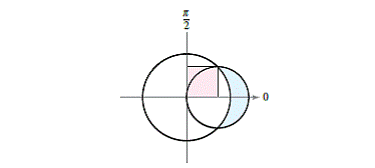Chapter 10.5, Problem 81E

Chapter
Section
Textbook Problem

# Area The larger circle in the figure is the graph of r = 1 . Find the polar equation of the smaller circle such that the shaded regions are equal.To determine

To Calculate: The polar equation of smaller circle such that shaded region are equal.

Explanation

Given:

The larger Circle in the figure is the graph of

r=1 shown in graph below:

Formula Used:

A=12ab[(upper)2(lower)2].dθ

Graph:

Larger Circle in the figure is graph of r=1

Smaller Circle has the equation r=acosθ

We have to find t he equation of smaller Circle such that shaded regions are equal

Two graphs intersect at θ=π4

And area of square that is shaded region is equal with shaded arc which is shown below

So area of shaded region is:

A=2(12)0π4[(acosθ)21].dθ

=0π4[(a2cos2θ)1].dθ

=[a22(θ+sin2θ2)θ]0π4

=[a22(π4+12)π4]

### Still sussing out bartleby?

Check out a sample textbook solution.

See a sample solution

#### The Solution to Your Study Problems

Bartleby provides explanations to thousands of textbook problems written by our experts, many with advanced degrees!

Get Started

#### Find more solutions based on key concepts